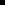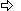### Push one or more Data Values

CimPairData provides a method called Push.

Push:Allows you to insert one or more pairs of X and Y data values through the end of the X and Y vectors. This is particularly useful if your series plots a continuous flow of data. An example of continuous flow of data is a function curve such as cosine of an incremental variable.Expects the variant type for the type of X and Y values

This variant type can be a single or array value of any of the following Basic types that result in a numeric value including:IntegerLongSingleDoubleDecimalBooleanByteVariantString

Example. Push One or More Data Values

You have a series whose X and Y values are the following:

 X 5 10 15 20 25 30 Y 22 17 9 -7 22 41

You push the following X and Y values:

 X 35 40 45 50 Y 59 37 21 6

The series X and Y values are now the following:

 X 25 30 35 40 45 50 Y 22 41 59 37 21 6

The first four pairs of XY values have been removed from the series because the size of the X and Y vectors is fixed.

Scripting examples for setting single and array values include:

Example 1. Push values into a Series

Series name: Series 1

Script purpose: Push an integer and a double value into Series 1

Procedure:

1. Open the CimEdit Properties dialog box for the XY Plot control object.

2. Display the Edit Script tab.

3. Write the following Basic excerpt within a subroutine.

' Create an integer and a double value:

Dim x as Integer

x = 7

Dim y as Double

Y = 22.74

' Push the data into the series object:

cimOleObj.Series("Series 1").Data.Push x, y

Example 2. Push Array Values into a Series

Series name: Series 1

Script purpose: Create an array of variant values and an array of double values and push them into Series 1

Procedure:

1. Open the CimEdit Properties dialog box for the XY Plot control object.

2. Display the Edit Script tab.

3. Write the following Basic excerpt within a subroutine.

' Create an array of variant values:

Dim x(4) As Variant

x(0) = 1

x(1) = Null

x(2) = 4.78

x(3) = Empty

x(4) ="5.78"

' Create an array of random double values:

Randomize

Dim y(4) As Double

For i = 0 To 4

y(i) = Random(0, 50)

Next i

' Push the data into the series object:

cimOleObj.Series("Series 1").Data.Push x, y

 Scripting examples for XY plots.